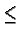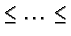CSE 5311 Fall 1999

Quiz #2

1.
(10 points.) Show how Quicksort can be made to run in O(n lg n) time in the worst case by making use of an order statistics algorithm.

Use the worst-case linear-time median finding algorithm to pick the pivot in the partitioning step of Quick sort. This guarantees an equal split in linear time, so the recurrence is T(n) = 2T(n/2) + Theta(n) = Theta(nlgn).

2.
(10 points.) Consider the following variant of insertion sort: for 2in, to insert the key A[i] among AA[i-1], perform a binary search to find the correct position for A[i].

How many key comparisons would be performed in the worst case?

We performlg i comparisons when inserting key i+1. The total number of comparisons in the worst case is.